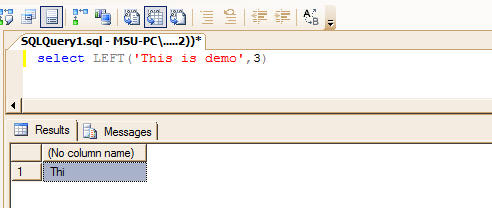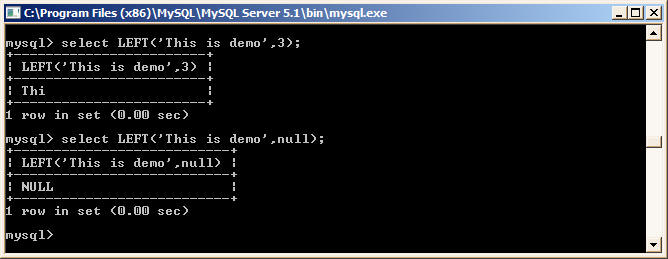﻿ LEFT String Function in MSSQL and it's equivalent in MySQL# LEFT function in MSSQL and MySQL

The LEFT function returns the specified number of characters from left in a given string. It functionality is same in both MSSQL and MySQL

## MSSQL

Syntax

`LEFT(String,n) `

Where

string:  Is the characters string from which you want to retrieve leftmost characters
n       :   Is the number of characters you want to retrieve

Example

`select LEFT('This is demo',3)`
```----------------------
Thi```## MySQL

It's similar to MSSQL LEFT function.

Example

```mysql> select LEFT('This is demo',3);
+------------------------+
| LEFT('This is demo',3) |
+------------------------+
| Thi |
+------------------------+
1 row in set (0.00 sec)

mysql>
```
``
` `

Back to Converting Functions from MSSQL to MySQL

` `